• 综合
• 股票
• 板块
• 嘉宾
• 课程
• 基金
• 经理
• 说说
• 掌评
• 消息# 韩永辉

VIP近日，国家发展改革委、民航局印发了《推进京津冀民航协同发展实施意见》。针对发展目标，《意见》提出，到2020年，北京新机场建成投入使用，京津冀机场群协同发展水平显著提升，整体服务水平、智能化水平、运营管理力争达到国际先进水平。到2030年，将打造形成分工合作、优势互补、空铁联运、协同发展的世界级机场群。

而在12月18日，在国家发改委新闻发布会上，发改委新闻发言人孟玮这样介绍了京津冀世界级机场群建设的相关情况。“京津冀世界级机场群，直接服务于京津冀世界级城市群的功能定位和发展要求，与世界一流航空枢纽相比，主要优势可以用’综合保障能力’和‘综合交通枢纽’这12个字概括。”

孟玮说，关于“综合保障能力”，也就是通过加快北京新机场建设，优化首都机场航线结构，完善首都机场服务功能，显著提升北京地区民航运输保障能力和辐射带动作用；通过改扩建天津滨海机场，建设我国国际航空物流中心，显著增强天津滨海机场区域枢纽作用。

另外通过改扩建石家庄正定机场，充分发挥正定机场比较优势，显著增强其对周边的集聚辐射作用。在此基础上，构建京津冀三省市航空枢纽协作机制，推进三地机场协同运行和联合管理，从而全面提升京津冀民用航空机场的综合保障能力。

关于“综合交通枢纽”，也就是在打造“轨道上的京津冀”同时，通过加强京津冀地区民用运输机场与轨道交通的衔接和融合，加快实现主要机场之间以及与周边重要城镇和功能区通过轨道交通快速连接，打造京津冀区域综合交通枢纽，推动空铁联程联运发展，从而全面提升空地一体化发展水平。

除了此次的意见外，国家发展和改革委员会早在今年3月15日就透露，《全国民用运输机场布局规划》日前已经正式印发。根据《规划》，到2020年，中国内地运输机场数量达到260个左右，到2025年新增机场136个，形成京津冀、长三角地区和珠三角地区三大世界级机场群，而此次的京津冀世界级机场仅仅是率先建设的国内三大世界级机场群之一。

业内人士分析指出，京津冀建设世界级机场群的重中之重是构建“通达全国、辐射全球”的国际航空交通体系，并充分发挥北京两大国际枢纽机场的“国之重器”作用。其总体目标不仅是服务于京津冀建设“以首都为核心的世界级城市群”和北京建设“四个中心”的战略目标，更是在“一带一路”国家战略中充分发挥着作为国际交通中心的核心动力源作用和国际枢纽节点作用。

另外随着京津冀世界级机场的建设，不仅可以缓解交通压力，更巩固了北京作为我国政治中心的纽带作用。受益于机场的建设，随着此次政策的落实，在政策的鼎力支持下，A股中金隅股份（601992）、冀东水泥（000401）、北京城建（600266）、首开股份（600376）、空港股份（600463）等概念股有望受益。

2017-12-18 15:40:05 展开全文 互动详情 296人气

2017-10-26 15:40:02 展开全文 互动详情 547人气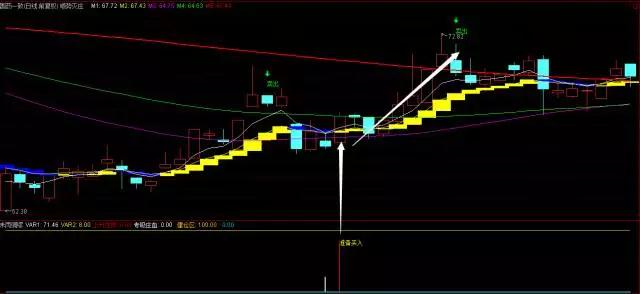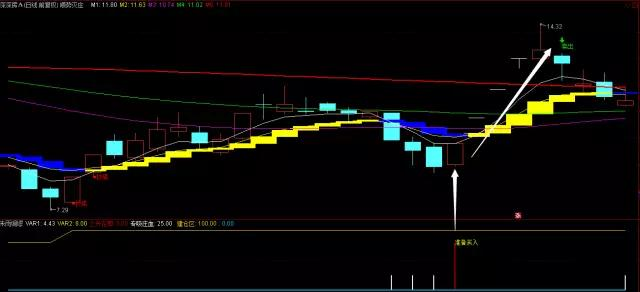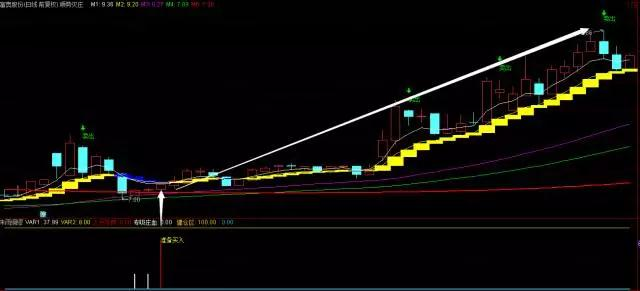1、顺势灭庄主图用法：

2、未雨绸缪副图用法：

3、二个指标配合使用说明：

“顺势灭庄”指标源码（主图）：

JJ:=(CLOSE+HIGH+LOW)/3;

A:=EMA(JJ,10);

B:=REF(A,1);

STICKLINE(A>B,A,B,5,0),COLORYELLOW;

STICKLINE(A<B,A,B,5,0),COLORBLUE;

M1:EMA(CLOSE,5);

M2:EMA(CLOSE,10);

M3:EMA(M2,30);

M4:EMA(M2,55);

VAR1:=EMA(M3,55);

M5:EMA(M4,81), COLORRED,LINETHICK2;

VAR2:=(REF(HIGH,1)+REF(LOW,1))/2;

VAR3:=MA(CLOSE,21)+2*STD(CLOSE,21);

DRAWICON(CROSS(VAR2,VAR3),HIGH*1.035,2);

DRAWTEXT(CROSS(VAR2,VAR3),HIGH*1.02,'卖出'), COLORGREEN;

VAR4:=REF(CLOSE,1);

VAR5:=SMA(MAX(CLOSE-VAR4,0),7,1)/SMA(ABS(CLOSE-VAR4),7,1)*100;

VAR6:=CROSS(79,VAR5);

VAR7:=FILTER(VAR6,4);

DRAWICON(VAR7,HIGH*1.035,2);

DRAWTEXT(VAR7,HIGH*1.02,'卖出'), COLORGREEN;

A7:=(2*C+H+L)/4;

VAR10:=LLV(LOW,34);

VAR11:=HHV(HIGH,34);

SK:=EMA((A7-VAR1)/(VAR2-VAR1)*100,7);

SD:=EMA(0.667*REF(SK,1)+0.333*SK,5);

DRAWTEXT(IF(COUNT(CLOSE<REF(CLOSE,1),8)/8>6/10 AND VOL>=1.5*MA(VOL,5) AND

COUNT(SK>=SD,3) AND REF(LOW,1)=LLV(LOW,120),1,0),LOW,'●买进') ,COLORRED;

DRAWTEXT(IF(COUNT(CLOSE<REF(CLOSE,1),13)/13>6/10 AND

COUNT(SK>SD,6) AND REF(LOW,5)=LLV(LOW,120) AND REF(CLOSE>=OPEN,4) AND

REF(CLOSE>OPEN,3) AND REF(CLOSE>OPEN,2) AND REF(OPEN>CLOSE,1) AND

OPEN>REF(CLOSE,1),1,0),LOW,'●买进'), COLORRED;

D:=MA(CLOSE,80)-MA(CLOSE,10)/3;

DRAWTEXT(IF(CLOSE<D AND VOL/240>MA(VOL,5)/240 AND CLOSE>REF(CLOSE,1)*1.08

AND CLOSE<MA(CLOSE,13)*1.3,1,0),LOW,'●抄底'),COLORRED;

“未雨绸缪”指标源码（副图）：

N:=5;

VAR1:4*SMA((CLOSE-LLV(LOW,N))/(HHV(HIGH,N)-LLV(LOW,N))*100,5,1)-

3*SMA(SMA((CLOSE-LLV(LOW,N))/(HHV(HIGH,N)-LLV(LOW,N))*100,5,1),3.2,1),COLORYELLOW,LINETHICK0;

VAR2:8,COLORGREEN,LINETHICK0;

DRAWTEXT(CROSS(VAR1,VAR2),80,'准备买入'),COLOR00FFFF;

VARO5:=LLV(LOW,27);

VARO6:=HHV(HIGH,34);

VARO7:=EMA((CLOSE-VARO5)/(VARO6-VARO5)*4,4)*25;

0,LINETHICK2 ,COLORFFCC00;

“未雨绸缪”指标源码（选股）：

N:=5;

VAR1:=4*SMA((CLOSE-LLV(LOW,N))/(HHV(HIGH,N)-LLV(LOW,N))*100,5,1)-

3*SMA(SMA((CLOSE-LLV(LOW,N))/(HHV(HIGH,N)-LLV(LOW,N))*100,5,1),3.2,1),COLORYELLOW,LINETHICK0;

VAR2:=8;

XG:CROSS(VAR1,VAR2);

2017-07-06 17:12:18 展开全文 互动详情 522人气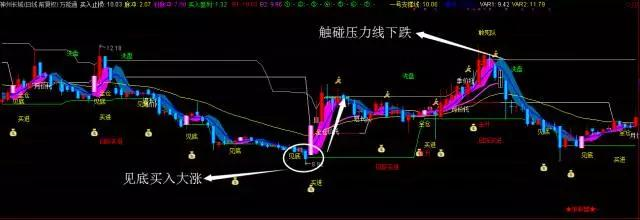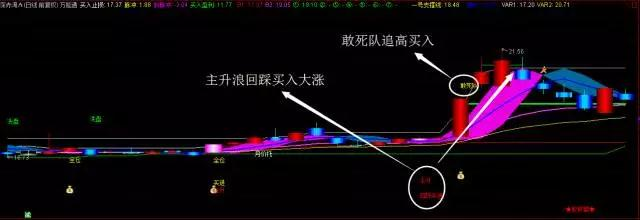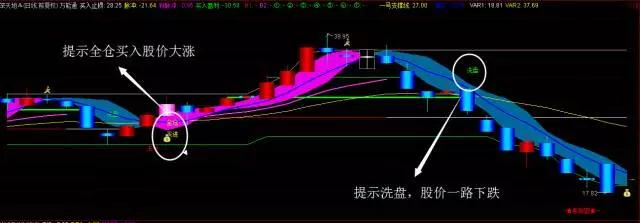1、该指标包含价托，买卖点，敢死队操作，庄家洗盘等等，首先韩老师解释一下何为价托。

2、5日均价线从下向上穿越10日均价线所形成的结点；5日均价线从下向上穿越20日均价线所形成的结点；10日均价线从下向上穿越20日均价线所形成的结点；由这三个结点组成一个封闭的三角形,这个三角形就称为“价托“。

3、三角形“托”在底部形态中是否有普遍性，考证后发现绝大多数个股都有这个规律，而在长期下跌的末端，这种三角形托几乎成了底部的代名词。

4、月价托指由5日、10日、20日均价线组成；该组合较为敏感,对中长期底部的提示作用较弱，对阶段性短期底部的提示有一定的参考作用；

5、季价托指由20日、40日、60日均价线组成；该组合的稳定度较好，对中长期底部的提示作用较好，但对阶段性短期底部的提示作用稍显迟钝；

6、短长结合的价托指由5日、10日、60日均价线组成；该组合既有一定的稳定度，也有一定的灵敏度；

7、指标中白色的为压力线，绿色的为支撑线。当股价达到或接近白色线时，我们就应该减仓或出货控制风险。

8、指标中紫红区域的为上升趋势线，蓝色区域为下降趋势线。无论买点何时提示，均需要在个股处于红色区域即上升趋势线中介入。另外指标其他买卖点提示都比较明显，大家结合实际情况操作即可哦！

A0:=(L+H+C*2)/4;

A1:=EMA(A0,14)COLORYELLOW,LINETHICK2;

A1X:=(A1-REF(A1,1))/REF(A1,1)*100,NODRAW;

A2X:=(EMA(A0,20)-REF(EMA(A0,20),1))/REF(EMA(A0,20),1)*100;

G1:=BARSLAST(CROSS(A1X,0));

DRAWTEXT_FIX(1,0.88,5,0,'~★股联盟★~'),COLORRED;

Y1:=REF(A0,G1)*1.1,COLORGREEN;

Y2:=REF(A0,G1)*1.2,COLORGREEN;

Y3:=REF(A0,G1)*1.3,COLORGREEN;

Y4:=REF(A0,G1)*1.4,COLORGREEN;

Y5:=REF(A0,G1)*1.5,COLORGREEN;

Y6:=REF(A0,G1)*1.6,COLORGREEN;

Y7:=REF(A0,G1)*1.7,COLORGREEN;

B1:IF(A1X>=0,买入止损,DRAWNULL),COLORRED,LINETHICK1;

B2:IF(A1X>=0,A1,DRAWNULL),COLORFF33FF,LINETHICK2;

①:IF(H>=Y1,Y1,DRAWNULL),COLORGREEN,LINETHICK3;

②:IF(H>=Y2,Y2,DRAWNULL),COLORGREEN;

③:IF(H>=Y3,Y3,DRAWNULL),COLORGREEN,CIRCLEDOT;

④:IF(H>=Y4,Y4,DRAWNULL),COLORGREEN;

⑤:IF(H>=Y5,Y5,DRAWNULL),COLORGREEN;

⑥:IF(H>=Y6,Y6,DRAWNULL),COLORGREEN;

⑦:IF(H>=Y7,Y7,DRAWNULL),COLORGREEN;

JX1:=MA(C,5);

JX2:=MA(C,10);

JX3:=MA(C,20);

JTTJ1:=BARSLAST(CROSS(JX1,JX2));

JTTJ2:=BARSLAST(CROSS(JX1,JX3));

JTTJ3:=BARSLAST(CROSS(JX2,JX3));

JTTJ4:=JTTJ1>=JTTJ2;

JTTJ5:=JTTJ2>=JTTJ3;

JTTJ6:=JTTJ3=0;

JX4:=MA(C,40);

JX5:=MA(C,60);

JTTJ7:=BARSLAST(CROSS(JX3,JX4));

JTTJ8:=BARSLAST(CROSS(JX3,JX5));

JTTJ9:=BARSLAST(CROSS(JX4,JX5));

JTTJ10:=JTTJ7>=JTTJ8;

JTTJ11:=JTTJ8>=JTTJ9;

JTTJ12:=JTTJ9=0;

JTTJ13:=BARSLAST(CROSS(JX1,JX2));

JTTJ14:=BARSLAST(CROSS(JX1,JX5));

JTTJ15:=BARSLAST(CROSS(JX2,JX5));

JTTJ16:=JTTJ13>=JTTJ14;

JTTJ17:=JTTJ14>=JTTJ15;

JTTJ18:=JTTJ15=0;

BOLL:=MA(C,20);

UPPER:=BOLL+2*STD(C,20);

LOWER:=BOLL-2*STD(C,20);

DRAWTEXT(大黑马 AND C>MA(C,60) AND C>MA(C,90) AND C>MA(C,120) AND C>MA(C,250),H*1.03,'敢死队'),COLORYELLOW;

DRAWTEXT(月价托,L*0.98,'月价托'),COLORWHITE;

DRAWTEXT(季价托,H+0.05,'季价托'),COLORWHITE;

DRAWTEXT(短长托,L-0.1,'短长托'),COLORWHITE;

MAHL1:=100*((EMA((H+L)/2,3)-LLV(EMA((H+L)/2,5),30)-(EMA(H,20)-EMA(L,20)))  /(LLV(EMA((H+L)/2,5),30)-(EMA(H,20)-EMA(L,20))));

MAHL2:=IF(EMA(C,2)>回归斜率线A AND MAHL1>0,EMA(C,3),LLV(EMA((H+L)/2,5),30));{A}

MAHL3:=IF(EMA(C,2)>回归斜率线A AND MAHL1>0,LLV(EMA((H+L)/2,5),30),EMA(C,5));{A}

DRAWTEXT(CROSS(一号支撑线1,上线1),H*1.08,'洗盘'),COLOR00FF00;

BBI:=(MA(CLOSE,3)+MA(CLOSE,6)+MA(CLOSE,12)+MA(CLOSE,25))/4;

UPR:=BBI+3*STD(BBI,13),LINETHICK2;

DWN:=BBI-3*STD(BBI,13);

LC:=REF(CLOSE,1);

RSI:=SMA(MAX(CLOSE-LC,0),6,1)/SMA(ABS(CLOSE-LC),6,1)*100;

DRAWTEXT( CROSS(82,RSI) AND CLOSE<上线1 , HIGH*1.04,'减仓') ,COLORFF9966,LINETHICK2;

A7:=(2*C+H+L)/4;

DRAWTEXT( CROSS(上线1,上线) AND 上线1>操作,LOW *0.92,'买进' ),COLORYELLOW;

VAR1:LLV(A7,21);

VAR2:HHV(A7,30);

DRAWTEXT( CROSS(上线1,操作) AND 上线1<一号支撑线 AND 上线1<一号支撑线1 AND 上线1<上线 AND CLOSE>VAR1,LOW *0.98,'见底' ),COLORYELLOW;

YCX:=操作>=REF(操作,1);

H1:=MAX(MAX(上线,一号支撑线1),一号支撑线);

L1:=MIN(MIN(上线,一号支撑线1),一号支撑线);

DRAWTEXT(CROSS(上线1,一号支撑线1) AND 上线1>操作 ,LOW *0.98,'全仓' ),COLORYELLOW;

SK:=EMA((A7-VAR1)/(VAR2-VAR1)*100,7);

SD:=EMA(0.667*REF(SK,1)+0.333*SK,5);

M1:=1000*操作/一号支撑线<=1015 AND 1000*操作/一号支撑线>=975;

M2:=1000*一号支撑线1/一号支撑线<=1020 AND 一号支撑线1/一号支撑线>=980;

M3:=1000*上线/一号支撑线<=1015 AND 上线/一号支撑线>=985;

DRAWTEXT(IF(COUNT(CLOSE<REF(CLOSE,1),13)/13>6/10 AND

COUNT(SK>SD,6) AND REF(LOW,5)=LLV(LOW,120) AND REF(CLOSE>=OPEN,4) AND

REF(CLOSE>OPEN,3) AND REF(CLOSE>OPEN,2) AND REF(OPEN>CLOSE,1) AND

OPEN>REF(CLOSE,1),1,0),LOW*0.94,'买进') ,COLORYELLOW;

D:=MA(CLOSE,89)-MA(CLOSE,10)/3;

DRAWTEXT(IF(CLOSE<D AND VOL/240>MA(VOL,5)/240 AND CLOSE>REF(CLOSE,1)*1.08

AND CLOSE<MA(CLOSE,13)*1.3,1,0),LOW*0.88,'抄底'),COLORBROWN;

RSV:=(CLOSE-LLV(LOW,25))/(HHV(HIGH,25)-LLV(LOW,25))*100;

K:=SMA(RSV,3,1);

D1:=SMA(K,3,1);

J:=3*K-2*D1;

VAR3:=(2*CLOSE+HIGH+LOW)/3;{可将3该为4，信号少点}

VAR4:=EMA(EMA(EMA(VAR3,4),4),4);{可将3该为4，信号少点}

E:=(VAR4-REF(VAR4,1))/REF(VAR4,1)*100;

F:=MA(E,3)+0.03;

G:=MA(E,1);

DRAWICON(CROSS(F,G) AND J>65,HIGH*1.02,15),COLORGREEN;

DRAWICON(CROSS(G,F),LOW *0.9,9),COLORYELLOW;

DRAWTEXT(CLOSE>OPEN AND H1<C AND O<L1 AND YCX AND CROSS(上线1,操作) AND LOW>VAR1 AND 上线1>一号支撑线 AND J<90,LOW *0.9,'主升' ),COLORRED;

DRAWTEXT( CROSS(上线1,操作) AND M1 AND M2 AND M3,LOW *0.94,'买进' ),COLORYELLOW;

DRAWTEXT(CLOSE>OPEN AND 上线>一号支撑线1 AND 一号支撑线1>一号支撑线 AND CLOSE>MA(CLOSE,一号支撑线) AND 操作>=REF(CLOSE,操作) AND CROSS(上线1,操作) AND LOW>VAR1 AND J<90,LOW *0.9,'主升' ),COLORRED;

DRAWTEXT(CLOSE>OPEN AND 操作>上线 AND 上线>一号支撑线1 AND 一号支撑线1>一号支撑线 AND CROSS(上线1,操作) AND LOW>VAR1 AND J<90,LOW *0.86,'回踩买进' ),COLORRED;

DRAWTEXT(CLOSE>OPEN AND CROSS(上线,一号支撑线) AND LOW>VAR1 AND J<90,LOW *0.86,'回踩买进' ),COLORRED;

STICKLINE(CLOSE> REF(CLOSE,1) ,HIGH,LOW,0,0 ),COLORRED;

STICKLINE(C<O AND C>REF(C,1),C,O,3,0),COLORFFCC00;

STICKLINE(CLOSE> REF(CLOSE,1) AND C>O ,OPEN,CLOSE,3,0 ),COLOR000055;{红K};

STICKLINE(CLOSE> REF(CLOSE,1) AND C>O  ,OPEN,CLOSE,2.7,0 ),COLOR000077;{红K};

STICKLINE(CLOSE> REF(CLOSE,1) AND C>O  ,OPEN,CLOSE,2.1,0 ),COLOR000099;

STICKLINE(CLOSE> REF(CLOSE,1) ,OPEN,CLOSE,1.5,0 ),COLOR0000BB;

STICKLINE(CLOSE> REF(CLOSE,1) ,OPEN,CLOSE,0.9,0 ),COLOR0000DD;

STICKLINE(CLOSE> REF(CLOSE,1) ,OPEN,CLOSE,0.3,0 ),COLOR0000FF;

STICKLINE(CLOSE= REF(CLOSE,1) ,H,L,3,1 ),COLOR999999;

STICKLINE(CLOSE= REF(CLOSE,1) ,HIGH,LOW,0,0 ),COLORWHITE;

STICKLINE(CLOSE= REF(CLOSE,1) ,OPEN,CLOSE,3,0 ),COLOR555555;

STICKLINE(CLOSE= REF(CLOSE,1) ,OPEN,CLOSE,2.7,0 ),COLOR777777;

STICKLINE(CLOSE= REF(CLOSE,1) ,OPEN,CLOSE,2.1,0 ),COLOR999999;

STICKLINE(CLOSE= REF(CLOSE,1) ,OPEN,CLOSE,1.5,0 ),COLORBBBBBB;

STICKLINE(CLOSE= REF(CLOSE,1) ,OPEN,CLOSE,0.9,0 ),COLORDDDDDD;

STICKLINE(CLOSE= REF(CLOSE,1) ,OPEN,CLOSE,0.3,0 ),COLORFFFFFF;

STICKLINE(CLOSE< REF(CLOSE,1) ,HIGH,LOW,0,1 ),COLORCYAN;

STICKLINE(C<REF(C,1) AND C>O,C,O,3,0),COLORFFCC00;

STICKLINE(C<REF(C,1) AND C<O ,OPEN,CLOSE,3,0 ),COLOR990000;

STICKLINE(C<REF(C,1) AND C<O ,OPEN,CLOSE,2.7,0 ),COLORCC0000;

STICKLINE(CLOSE< REF(C,1) ,OPEN,CLOSE,2.1,0 ),COLORFF4400;

STICKLINE(CLOSE< REF(C,1) ,OPEN,CLOSE,1.5,0 ),COLORFF8800;

STICKLINE(CLOSE< REF(C,1) ,OPEN,CLOSE,0.9,0 ),COLORFFCC00;

STICKLINE(CROSS(J,D1) AND J<70,CLOSE,OPEN,3.2,0),COLORBB00FF;

STICKLINE(CROSS(J,D1) AND J<70,CLOSE,OPEN,2.7,0),COLORFF33FF;

STICKLINE(CROSS(J,D1) AND J<70,CLOSE,OPEN,2.1,0), COLORFF66FF;

STICKLINE(CROSS(J,D1) AND J<70,CLOSE,OPEN,1.5,0),COLORFF99FF;

STICKLINE(CROSS(J,D1) AND J<70,CLOSE,OPEN,0.9,0),COLORFFBBFF;

STICKLINE(CROSS(J,D1) AND J<70,CLOSE,OPEN,0.3,0),COLORFFDDFF;

2017-06-16 16:44:07 展开全文 互动详情 587人气

"红杏出墙"抓暴涨，把把必中心不慌！

1、 横盘时间长；

2、 均线粘合发散；

3、 一根突破均线的大阳线；

4、 成交量急剧持续放大；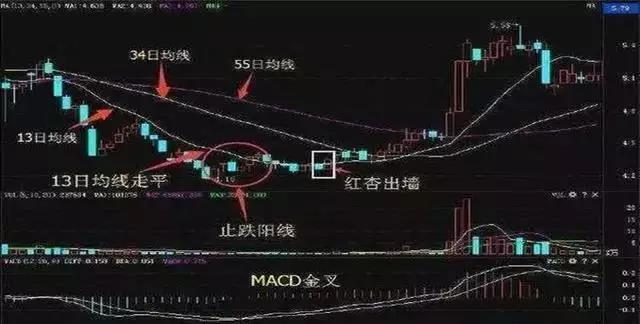1、在运用【红杏出墙】这个买点的时候，一定要注意13日均线是否走平，这是【红杏出墙】成败的关键。

2、股价在下跌的过程中。如果没有【红杏出墙】出现，股价就根本没有底部可言。

3、13日均线由跌趋走稳以后，股价一般要围绕着13日均线上下波动几天，而股价在13日均线上企稳后，k线多以小阳或中阳形态向上推进，显得很有节奏。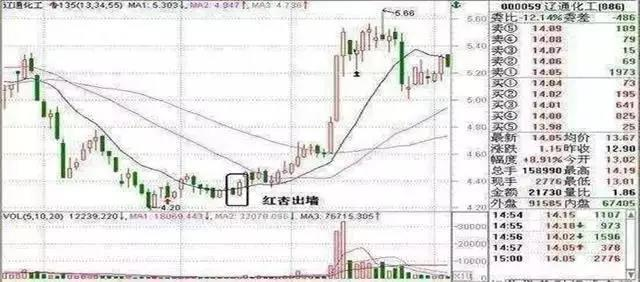1、红杏出墙形态出现当天，轻仓试探；

2、次日，盘中择低点介入。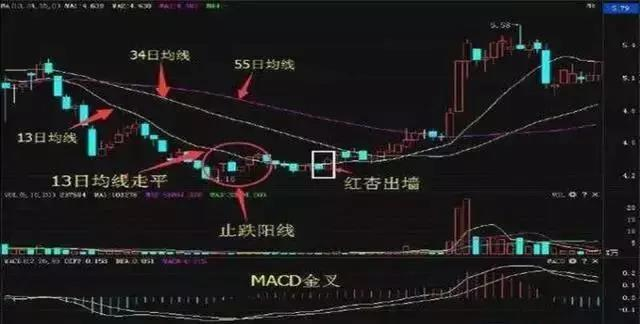2017-05-09 23:29:10 展开全文 互动详情 610人气

1、倒垂线主图：

M5:MA(C,5);

M10:MA(C,10);

M20:MA(C,20);

T:=BARSLAST(L>REF(L,1) AND REF(L,1)<REF(L,2));

SY:=H/MAX(C,O)*100;

XY:=MIN(C,O)/L*100;

BL:=SY/XY*100;

TJ:=BL>103.85 AND SY>105.05;

TJ1:=L>MAX(MAX(M5,M10),M20);

TJ2:=T=2 OR T=3;

XG:=TJ AND TJ1 AND TJ2;

DRAWICON(XG,L*0.95,1);

STICKLINE(XG,C,O,2,0),COLORYELLOW;

STICKLINE(XG,L,H,0,0),COLORYELLOW;

2、倒垂线副图：

M5:=MA(C,5);

M10:=MA(C,10);

M20:=MA(C,20);

T:=BARSLAST(L>REF(L,1) AND REF(L,1)<REF(L,2));

SY:=H/MAX(C,O)*100;

XY:=MIN(C,O)/L*100;

BL:=SY/XY*100;

TJ:=BL>103.85 AND SY>105.05;

TJ1:=L>MAX(MAX(M5,M10),M20);

TJ2:=T=2 OR T=3;

XG:=TJ AND TJ1 AND TJ2;

STICKLINE(XG,0,8,2,0),COLORYELLOW;

3、倒垂线选股：

M5:=MA(C,5);

M10:=MA(C,10);

M20:=MA(C,20);

T:=BARSLAST(L>REF(L,1) AND REF(L,1)<REF(L,2));

SY:=H/MAX(C,O)*100;

XY:=MIN(C,O)/L*100;

BL:=SY/XY*100;

TJ:=BL>103.85 AND SY>105.05;

TJ1:=L>MAX(MAX(M5,M10),M20);

TJ2:=T=2 OR T=3;

XG:TJ AND TJ1 AND TJ2;

2016-11-08 12:03:06 展开全文 互动详情 1125人气

2016-11-08 11:29:33 展开全文 互动详情 286人气

2016-11-07 21:41:38 展开全文 互动详情 504人气

2016-11-07 09:18:33 展开全文 互动详情 196人气

2016-05-23 10:23:30 展开全文 互动详情 1120人气

#### 实盘数据点掌实盘说明

• 点掌主题
• 点掌成长
• 点掌蓝筹

• 一周
• 一月
• 三月
• 六月
• 一年
• 创建以来
• 对标涨跌：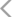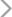• 手机号码：

请输入手机号码，您的个人信息严格保密，请放心

• 图形验证码：

请输入右侧图形验证码

• 短信验证码：

请输入接收的短信验证码

获取短信验证码
X

X

（一）按照“后台实名、前台自愿”原则，对注册用户进行真实身份信息认证，不得向未认证真实身份信息的用户提供跟帖评论服务。

（二）建立健全用户信息保护制度，收集、使用用户个人信息应当遵循合法、正当、必要的原则，公开收集、使用规则，明示收集、使用信息的目的、方式和范围，并经被收集者同意。

（三）对新闻信息提供跟帖评论服务的，应当建立先审后发制度。

（四）提供“弹幕”方式跟帖评论服务的，应当在同一平台和页面同时提供与之对应的静态版信息内容。

（五）建立健全跟帖评论审核管理、实时巡查、应急处置等信息安全管理制度，及时发现和处置违法信息，并向有关主管部门报告。

（六）开发跟帖评论信息安全保护和管理技术，创新跟帖评论管理方式，研发使用反垃圾信息管理系统，提升垃圾信息处置能力；及时发现跟帖评论服务存在的安全缺陷、漏洞等风险，采取补救措施，并向有关主管部门报告。

（七）配备与服务规模相适应的审核编辑队伍，提高审核编辑人员专业素养。

（八）配合有关主管部门依法开展监督检查工作，提供必要的技术、资料和数据支持。• 10牛
• 30牛
• 50牛
• 其它